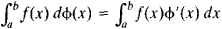# Stieltjes Integral

## Stieltjes integral

[′stēlt·yəs ‚int·ə·grəl]
(mathematics)
The Stieltjes integral of a real function ƒ(x) relative to a real function g (x) of bounded variation on an interval [a,b ] is defined, analogously to the Riemann integral, as a limit of a sum of terms ƒ(ai ) [g (xi ) - g (xi-1)] taken as partitions of the interval shrink. Denoted Also known as Riemann-Stieltjes integral.
McGraw-Hill Dictionary of Scientific & Technical Terms, 6E, Copyright © 2003 by The McGraw-Hill Companies, Inc.
The following article is from The Great Soviet Encyclopedia (1979). It might be outdated or ideologically biased.

## Stieltjes Integral

a generalization of the definite integral proposed in 1894 by T. Stieltjes. In this generalization, the limit of the Riemann sums ∑f(ξi)(xi – xi-1) is replaced by the limit of the sums ∑f(ξi) [Φ(xi) – Φ(xi-1)]. where the integrating function ϕ(x) is a function of bounded variation (seeVARIATION OF A FUNCTION). If ϕ(x) is differentiable, then the Stieltjes integral can be expressed in terms of the Riemann integral (if it exists):References in periodicals archive ?
In 2000, Dragomir (2) answered to the problem of approximating the Stieltjes integral [[integral].sub.a.sup.b]f(x)du(x) by the quantity [u(b) - u(a)]f (x), which is a natural generalization of the Ostrowski problem (3) analysed in 1937.
The text covers the Stieltjes integral, fundamental formulas, the moment problem, absolutely and completely monotonic functions, Tauberian theorems, the bilateral Laplace transform, inversion and representation problems for the Laplace transform, and the Stieltjes transform.
and integrating by parts in the Stieltjes integral [[integral].sub.a.sup.b] [PSI] (t) df (t), which exists, since f is of bounded variation and [PSI] is differentiable on (a, b).
If u and [phi] are two real-valued functions defined on the interval [a, b], then under some appropriate conditions (see ) we can define the Stieltjes integral (in the Riemann-Stieltjes sense)
The following theorem gives conditions under which we can take the limit of a sequence [([h.sub.n).sub.n[member of]N] of functions inside a Stieltjes integral.
Jankowski, Monotone iterative method to second order differential equations with deviating arguments involving Stieltjes integral boundary conditions, Dynam.
Thus, lower and upper bounds for the quantity [[rho].sub.[mu]] defined by (2.3) can be determined by replacing the Stieltjes integral in (2.13) by the Gauss and Gauss-Radau quadrature rules (3.14) and (3.15), respectively.
Li, "Positive solutions of second-order problem with dependence on derivative in nonlinearity under Stieltjes integral boundary condition," Electronic Journal of Qualitative Theory of Differential Equations, no.
Jankowski, Positive solutions for second order impulsive differential equations involving Stieltjes integral conditions, Nonlinear Anal., 74:3775-3785, 2011.
where [D.sup.[alpha].sub.0+] is the Riemann-Liouville fractional derivative, n - 1 < [alpha] [less than or equal to] n, n [greater than or equal to] 2,[lambda]X[z] = [[integral].sup.1.sub.0] z(t) dA (t) is a linear functional on C[0, 1] given by a Stieltjes integral with A representing a suitable function of bounded variation, and dA can be a signed measure.
The Integration by parts Corollary 27 yields a new representation theorem without using Stieltjes integral, which we shall establish next.
Wu, "Positive Solutions for Second-Order Singular Semipositone Differential Equations Involving Stieltjes Integral Conditions," Abstract and Applied Analysis, vol.

Site: Follow: Share:
Open / Close